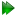Subsections

structure.create_diff_tensor_pdbSynopsis

Create a PDB file to represent the diffusion tensor.

Defaults

structure.create_diff_tensor_pdb(scale=1.8e-06, file=`tensor.pdb', dir=None, force=False)

Keyword arguments

scale: Value for scaling the diffusion rates.

file: The name of the PDB file.

dir: The directory to place the file into.

force: A flag which, if set to True, will overwrite the any pre-existing file.

Description

This creates a PDB file containing an artificial geometric structure to represent the diffusion tensor. A structure must have previously been read into relax. The diffusion tensor is represented by an ellipsoidal, spheroidal, or spherical geometric object with its origin located at the centre of mass (of the selected residues). This diffusion tensor PDB file can subsequently read into any molecular viewer.

There are four different types of residue within the PDB. The centre of mass of the selected residues is represented as a single carbon atom of the residue `COM'. The ellipsoidal geometric shape consists of numerous H atoms of the residue `TNS'. The axes of the tensor, when defined, are presented as the residue `AXS' and consist of carbon atoms: one at the centre of mass and one at the end of each eigenvector. Finally, if Monte Carlo simulations were run and the diffusion tensor parameters were allowed to vary then there will be multiple `SIM' residues, one for each simulation. These are essentially the same as the `AXS' residue, representing the axes of the simulated tensors, and they will appear as a distribution.

As the Brownian rotational diffusion tensor is a measure of the rate of rotation about different axes - the larger the geometric object, the faster the diffusion of a molecule. For example the diffusion tensor of a water molecule is much larger than that of a macromolecule.

The effective global correlation time experienced by an XH bond vector, not to be confused with the Lipari and Szabo parameter τ_e, will be approximately proportional to the component of the diffusion tensor parallel to it. The approximation is not exact due to the multiexponential form of the correlation function of Brownian rotational diffusion. If an XH bond vector is parallel to the longest axis of the tensor, it will be unaffected by rotations about that axis, which are the fastest rotations of the molecule, and therefore its effective global correlation time will be maximal.

To set the size of the diffusion tensor within the PDB frame the unit vectors used to generate the geometric object are first multiplied by the diffusion tensor (which has the units of inverse seconds) then by the scaling factor (which has the units of second Åand has the default value of 1.8e-6 s.Angstrom). Therefore the rotational diffusion rate per Åis equal the inverse of the scale value (which defaults to 5.56e5 s^-1.Angstrom^-1). Using the default scaling value for spherical diffusion, the correspondence between global correlation time,diffusion rate, and the radius of the sphere for a number of discrete cases will be:

Please see Table 17.26 on page.

 τm (ns)(s^-1) Radius (Å) 1 1.67e8 300 3 5.56e7 100 10 1.67e7 30 30 5.56e6 10

The scaling value has been fixed to facilitate comparisons within or between publications, but can be changed to vary the size of the tensor geometric object if necessary. Reporting the rotational diffusion rate per Åwithin figure legends would be useful.

To create the tensor PDB representation, a number of algorithms are utilised. Firstly the centre of mass is calculated for the selected residues and is represented in the PDB by a C atom. Then the axes of the diffusion are calculated, as unit vectors scaled to the appropriate length (multiplied by the eigenvalue,,,,, oras well as the scale value), and a C atom placed at the position of this vector plus the centre of mass. Finally a uniform distribution of vectors on a sphere is generated using spherical coordinates. By incrementing the polar angle using an arccos distribution, a radial array of vectors representing latitude are created while incrementing the azimuthal angle evenly creates the longitudinal vectors. These unit vectors, which are distributed within the PDB frame and are of 1 Åin length, are first rotated into the diffusion frame using a rotation matrix (the spherical diffusion tensor is not rotated). Then they are multiplied by the diffusion tensor matrix to extend the vector out to the correct length, and finally multiplied by the scale value so that the vectors reasonably superimpose onto the macromolecular structure. The last set of algorithms place all this information into a PDB file. The distribution of vectors are represented by H atoms and are all connected using PDB CONECT records. Each H atom is connected to its two neighbours on the both the longitude and latitude. This creates a geometric PDB object with longitudinal and latitudinal lines.

The relax user manual (PDF), created 2020-08-26.